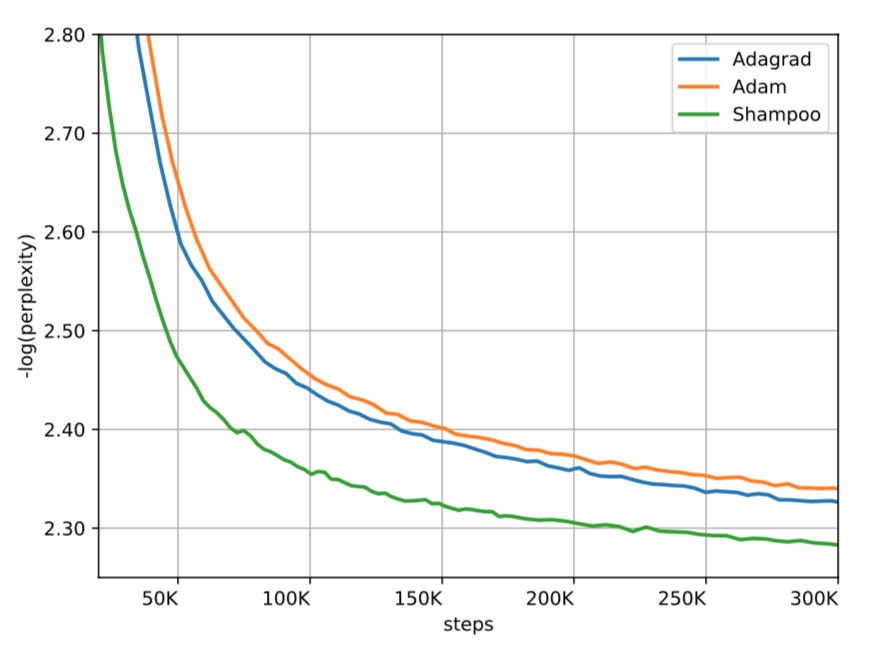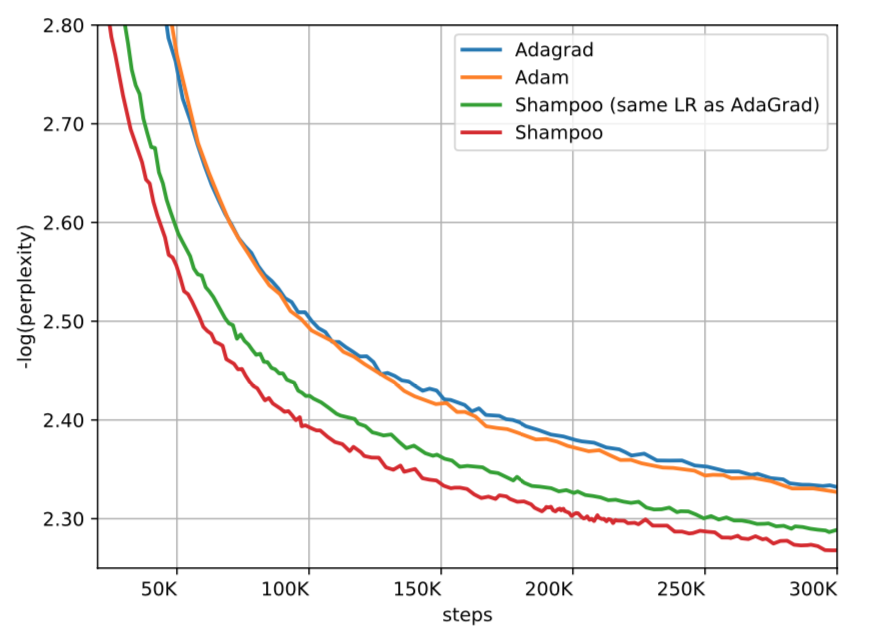# 实用二阶优化

## Shampoo (2018)

$$L_t = L_{t-1} + \triangledown f(W_t) \triangledown f(W_t)^T, L_0 = 0$$ $$R_t = R_{t-1} + \triangledown f(W_t)^T \triangledown f(W_t), R_0 = 0$$ $$W_{t+1} = W_t - \eta L_t^{-1/4} \triangledown f(W_t)_t R_t^{-1/4}$$

## Challenges and Limitations

1. 现有设备面向一阶优化算法，算力与内存低；
2. Shampoo 需要立方级的计算，如何分摊？
3. 另外需要平方级的空间，在大型网络的应用中必须分片计算，如何避免过于影响精度？
4. 硬件加速普遍只支持 8/16 位的精度，但 Shampoo 改需要双精度；
5. API 层面如 TensorFlow 支持不好

## 计算瓶颈$L_T^{-1/4}$ 是最大瓶颈

$$A = Q \Sigma Q^T = (Q \sqrt{\Sigma}) (Q \sqrt{\Sigma})^T$$

Q 是正交矩阵，$\Sigma$ 是对角矩阵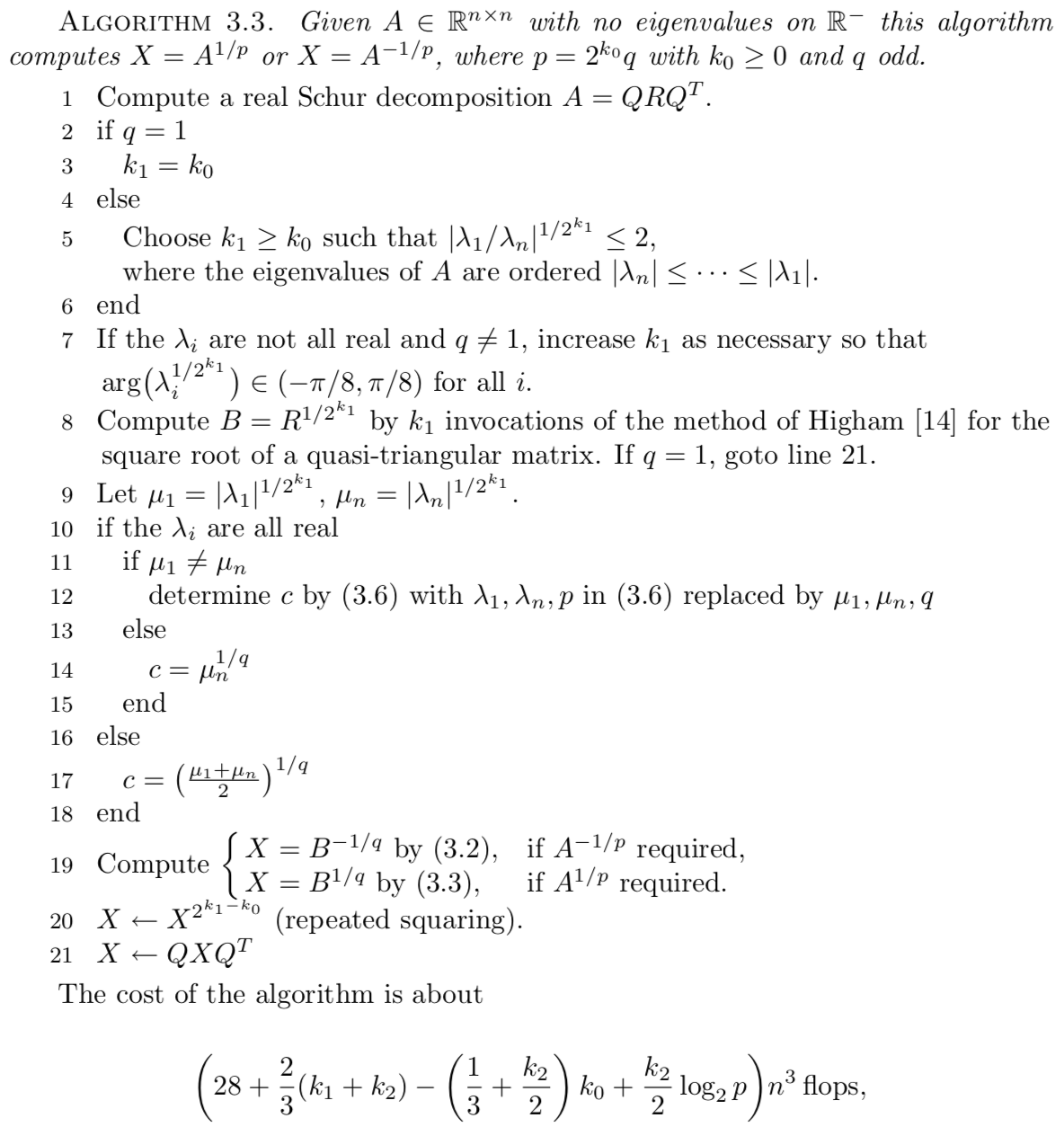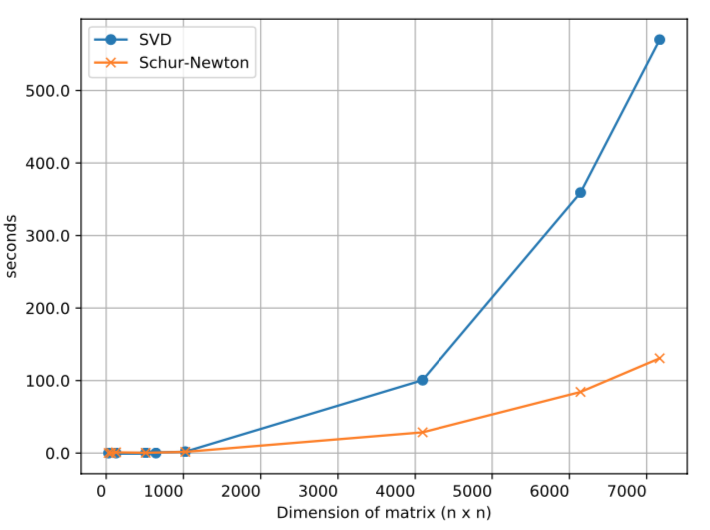## Other Contributions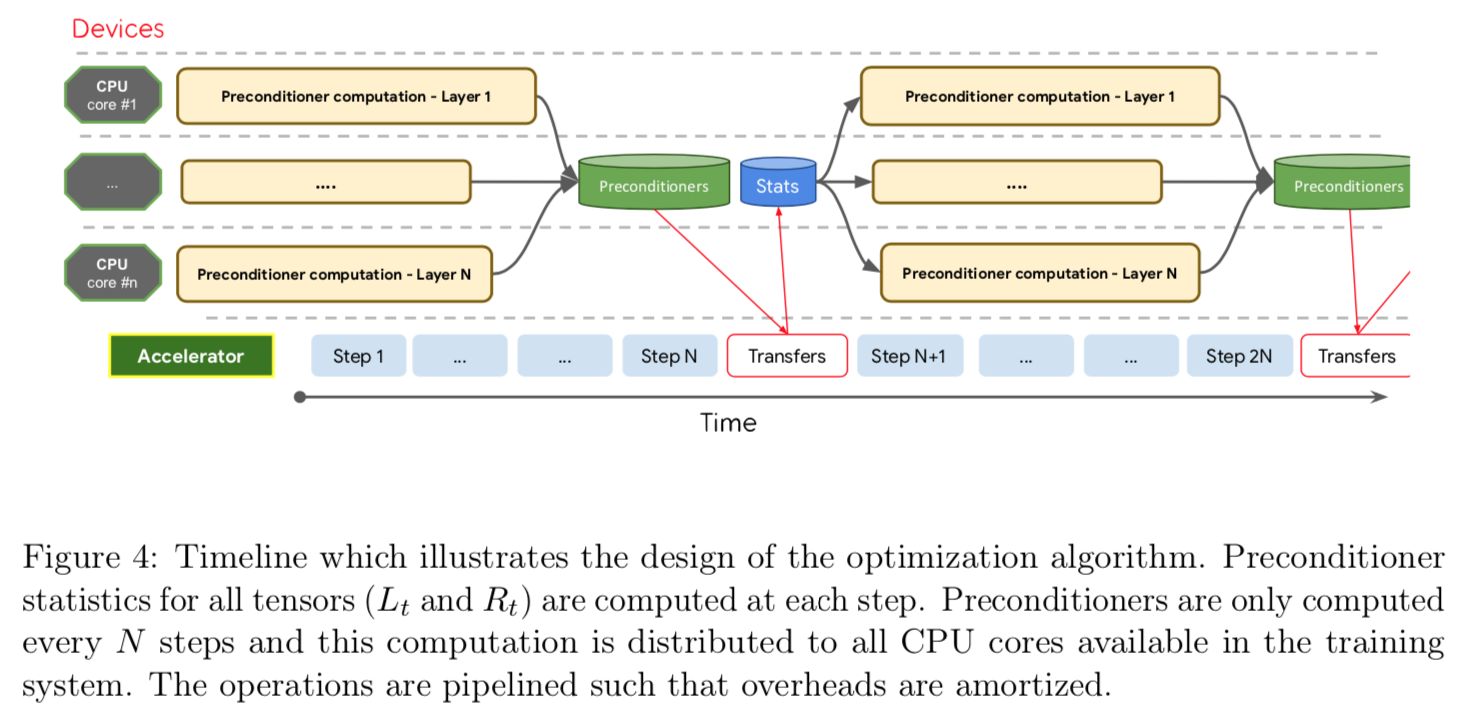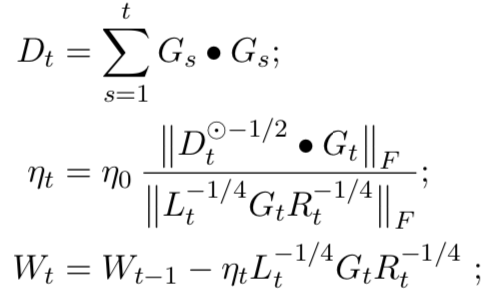## 整体效果